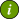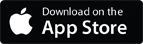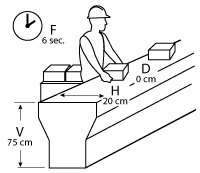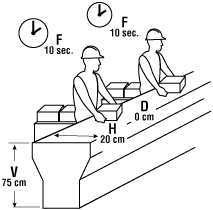### OSH Answers Fact SheetsEasy-to-read, question-and-answer fact sheets covering a wide range of workplace health and safety topics, from hazards to diseases to ergonomics to workplace promotion. MORE ABOUT >Search all fact sheets:

Type a word, a phrase, or ask a question

# Frequency Factor

## How can the frequency factor affect the weight limit?

Example: A worker lifts 10 kg boxes from the conveyor to the cart ten times every minute for two-hours.To calculate the recommended weight limit (RWL) for the task:

• Determine the weight of the load.

Weight equals 10 kg

H (Horizontal Distance)- 20 cm
V (Vertical Distance) - 75 cm
D (Lifting/ carrying Distance) - 0 cm
F (Frequency) - 6 sec over 1 hour, standing
A (Angle) - 90°
C (Coupling/quality of grip) - fair, standing

The revised NIOSH Lifting Equation is: 23 kg x HM x VM x DM x AM x FM x CM = RWL

23 kg x 1.00 x 0.93 x 1.00 x 0.71 x 0.13 x 1.00 = 1.97 kg

* In this example, the vertical distance is 75 cm. From the tables, the vertical multiplier (VN) was greater than 70 cm (value of 0.99), so the value of 0.93 was selected (lifting distance of 70 cm) to be more protective.

• Compare weight of the load against the calculated Recommended Weight Limit for the task. The weight of 10 kg is much larger than the recommended weight limit of 1.97 kg.

Conclusion

The weight of the load is 10 kg, which is higher than the calculated recommended weight limit of 1.97 kg.

Therefore, this task is likely to increase the risk of a lifting related injury.

### Recommendations

• Assess which of components contribute more to the risk. Refer to Assessing Relevant Handling Factors.
• the critical factor is FM for the frequency of lifting and duration of task.
• Shorten the frequency of lifting by:
• reducing the frequency of incoming boxes in half, or
• shorten the time of the task to 1 hour.The revised NIOSH Lifting Equation is: 23 kg x HM x VM x DM x AM x FM x CM = RWL

23 kg x 1.00 x 0.93 x 1.00 x 0.71 x 0.75 x 1.00 = 9.90 kg

* In this example, the vertical distance is 75 cm. From the tables, the vertical multiplier (VN) was greater than 70 cm (value of 0.99), so the value of 0.93 was selected (lifting distance of 70 cm) to be more protective.

• Compare weight of the box against Recommended Weight Limit for redesigned task.

The weight of the load at 10 kg is now similar to the calculated weight limit of 9.90 kg.

Further improvement can be achieved by reducing the weight of the object.

Therefore, while most workers can likely safely perform the task, the work should be monitored for the development of injuries.

Document last updated on April 27, 2021